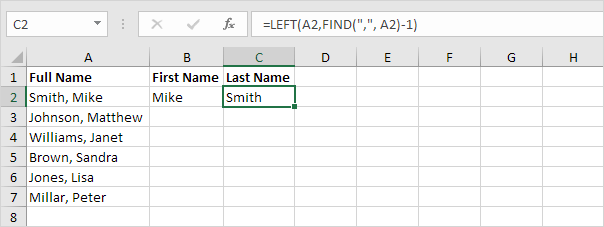# Separate Strings

This example teaches you how to separate strings in Excel.The problem we are dealing with is that we need to tell Excel where we want to separate the string. In case of Smith, Mike the comma is at position 6 while in case of Williams, Janet the comma is at position 9.

1. To get the first name, use the formula below.Explanation: to find the position of the comma, use the FIND function (position 6). To get the length of a string, use the LEN function (11 characters). =RIGHT(A2,LEN(A2)-FIND(“,”,A2)-1) reduces to =RIGHT(A2,11-6-1). =RIGHT(A2,4) extracts the 4 rightmost characters and gives the desired result (Mike).

2. To get the last name, use the following formula.Explanation: to find the position of the comma, use the FIND function (position 6). =LEFT(A2,FIND(“,”, A2)-1) reduces to =LEFT(A2,6-1). =LEFT(A2,5) extracts the 5 leftmost characters and gives the desired result (Smith).

3. Select the range B2:C2 and drag it down.